# Marvin tools tutorial

Back to MaNGA tutorials

The goal of this introductory tutorial will be to show you the basics of loading and visualizing MaNGA DAP products using Marvin from start-to-finish. It assumes you’re using ipython or jupyter notebooks (recommended when using Marvin). If you're using Marvin for the first time, you'll want to follow the instructions to install the package and configure it to automatically download data.

Let’s first import the packages we’ll need and turn on interactive plotting:

import matplotlib.pyplot as plt
from marvin.tools.maps import Maps
import marvin.utils.plot.map as mapplot
from marvin.tools.image import Image
plt.style.use('seaborn-darkgrid')  # set matplotlib style sheet


If you notice that the plots from this tutorial do not always display automatically, you may need to explicitly turn on matplotlib interactive plotting:

plt.ion()


We will do a quick Map plot for the MaNGA object (plate-ifu) 7443-12703. This is the galaxy merger Mrk 848. You can see how this galaxy looks like in the SDSS image.

image = Image(plateifu='7443-12703')
image.plot()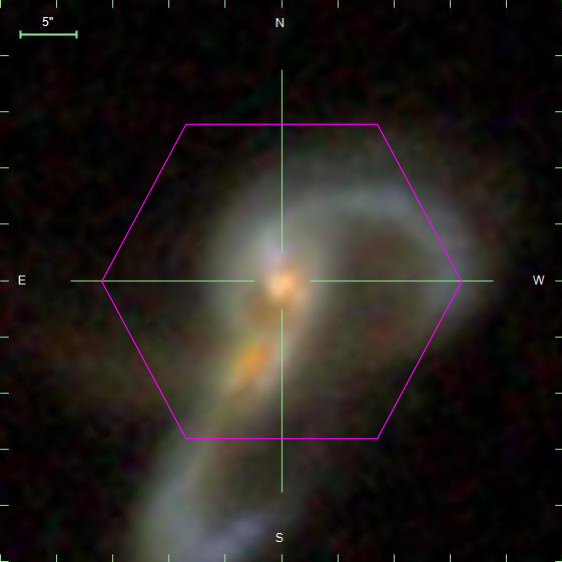SDSS three-color image of the MaNGA galaxy 7443-12703.

The first thing to do is to load the map file. Note that you do not need to have the map file, Marvin will access to the file remotely if it doesn't find it in your computer.

maps = Maps(plateifu='7443-12703')


This file contains several extensions. To see them all, type:

maps.datamodel


For a full description of these extensions, see the DAP documentation.

## Making an emission line map and applying masks

Let's make a simple map of Hα flux. For that, we just need to type:

ha = maps.emline_gflux_ha_6564


Now we can plot the map:

fig, ax = ha.plot()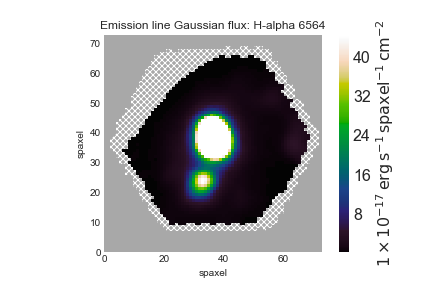Map of Hα emission.

Note that Marvin already masks the spaxels with unreliable measurements, excluding all regions that have a non-zero mask.

Now, let's use the same basic procedure to plot the ionized gas velocity field.

gas_vfield = maps.emline_gvel_ha_6564
fig, ax = gas_vfield.plot()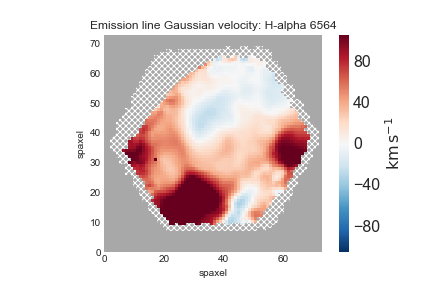An ionized gas velocity field.

Depending on your science, you may want to only examine regions with very well-detected emission lines. For that, we will be only interested in the spaxels where the line flux has S/N > 10:

mask_snr10 = (ha.snr < 10) * ha.pixmask.labels_to_value('DONOTUSE')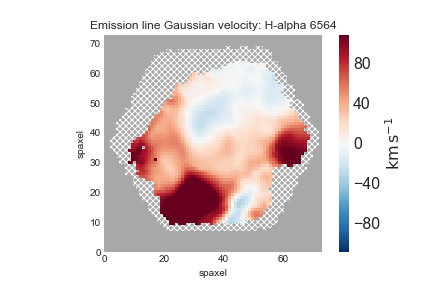An ionized gas velocity field limited to regions where the Hα flux has S/N>10.

An important note regarding gas velocity fields: The velocities of the emission lines are tied together, so for example, the velocity of the [OIII]-5007 line is the same as the H&alpha line, as are the uncertainties. You cannot reduce the uncertainty on the measured velocity by averaging the velocities of several lines together.

## Plotting stellar velocity dispersion

Next we'll make a map of the stellar velocity dispersion. First, we'll access the raw dispersion measurements:

disp_raw = maps.stellar_sigma


However, we need to correct the measured dispersion for the instrumental resolution, which is reported in the stellar_sigmacorr extension, though we don't need to access it because Marvin has a function do the correction. Let's apply the correction and plot the results (also removing masked values). The calculation below will ignore any points where the correction is larger than the measured dispersion:

disp_stars_corr = disp_raw.inst_sigma_correction()
disp_stars_corr.plot()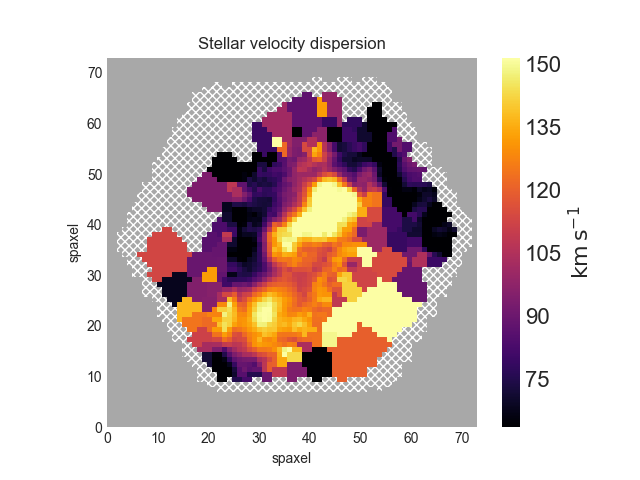Stellar velocity dispersion after correction for instrumental spectral resolution.

## Plotting spectral-index measurements

Next we'll show how to plot spectral indices and correct them for velocity dispersion. The spectral index measurements need to be corrected for velocity dispersion. Keep in mind that the way in which the corrections are applied depends on whether the units are angstroms or magnitudes. Hβ is in Angstroms:

hb_raw = maps.specindex_hb
corr = maps.specindex_corr_hb
hb_corr = hb_raw*corr
fig, ax = mapplot.plot(value=hb_corr.value, dapmap=hb_raw)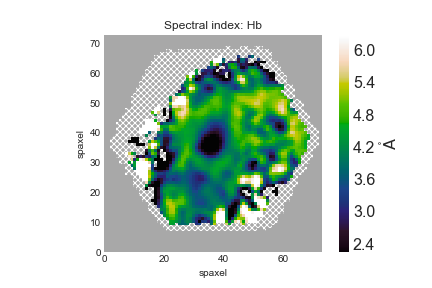Hβ spectral index measurement after applying the correction for velocity dispersion.

## Identifying unique bins

As explained in the corresponding DAP tutorial, the spaxels in a map can be organised in different bins depending on the measurement we are making. The BINID extension provides five different binid channels. With Marvin it is very easy to access the binid information for any of these channels. For instance, let us get the indices of the unique bins where stellar kinematics were fit for 7443-12703:

from marvin.tools.maps import Maps
import numpy
my_map = Maps('7443-12703', bintype='HYB10')
binid_kin = my_map.binid_stellar_continua
__, unique_indices = numpy.unique(binid_kin.value, return_index=True)


You can use the datamodel to get more information about the available properties. To access it, do my_map.datamodel.

Now, as in the DAP example, we can use these unique indices to plot the position of each unique bin and color-code it using the measured stellar velocity:

x = my_map.bin_lwskycoo_lum_weighted_on_sky_x.value.ravel()[unique_indices]
y = my_map.bin_lwskycoo_lum_weighted_on_sky_y.value.ravel()[unique_indices]

from matplotlib import pyplot
pyplot.scatter(x, y, c=v,  vmin=-150, vmax=150, cmap='RdBu', marker='.', s=30, lw=0,)
pyplot.colorbar()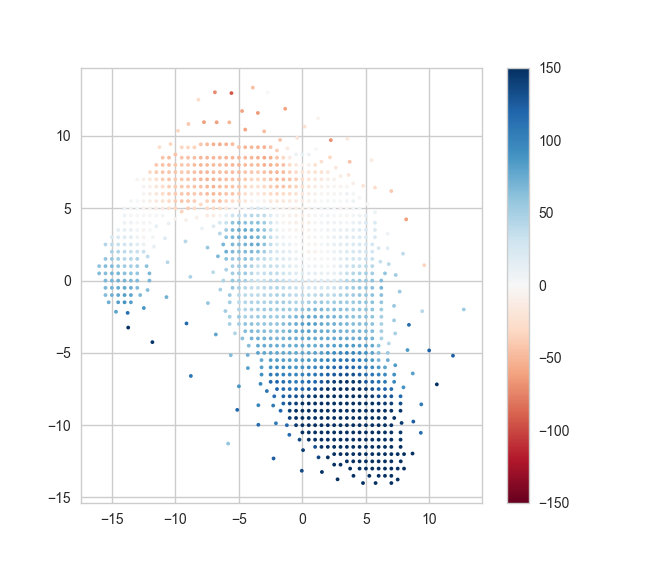Positions of each unique stellar velocity measurement from the binned spectra, color-coded by value.

Note how accessing the data using Marvin is much clearer and more readable!

## Extract a binned spectrum and its model fit

With Marvin is very simple to plot the flux and associated modelled quantities for a given bin or spaxel. Before you continue reading, make sure you understand the differences between HYB10 and VOR10 model cubes (go here for a more detailed explanation). Since HYB10 maps and modelcubes are recommended for most users, Marvin only allows remote access to that bintype. VOR10 data can be accessed with Marvin only in file mode.

Let us start by selecting the coordinates of the bin with the highest SNR

from marvin.tools.maps import Maps
my_map = Maps('7443-12703', bintype='HYB10')
snr = my_map.bin_snr
j, i = numpy.unravel_index(numpy.argmax(snr), snr.shape)


Now let us grab the bin associated with those coordinates

from marvin.tools.modelcube import ModelCube
my_modelcube = ModelCube('7443-12703', bintype='HYB10')
my_bin = my_modelcube[j, i]
print(my_bin)


The Marvin Bin allows us to access to all the DRP and DAP measurements for that bin. For HYB10, if we want to compare the full fit (stellar + emission line) with the original spectrum, we must use the DRP LOGCUBE file. To get the corresponding DRP spectrum

from marvin.tools.cube import Cube
my_drpcube = Cube('7443-12703')
drp_flux = my_drpcube[j, i].flux


Hint: While you can also do drp_flux = my_drpcube.flux[jj, ii], that will force Marvin to send the whole DRP cube over the API, which may take a long time depending on your connection. drp_flux = my_drpcube[jj, ii].flux ensures that only the desired spaxel is retrieved.

Now that we have all the information we need let us plot the flux and some model properties

ax = drp_flux.plot(show_std=True, n_sigma=3)
ax.lines.set_label('flux')  # A bit of black magic to set the legend label
ax.plot(my_bin.emline_fit.wavelength, my_bin.emline_fit.masked, color='m', lw=1, label='Emission lines')

ax.step(drp_flux.wavelength, resid, where='mid', color='0.5', lw=0.5,label='residuals')

ax.legend()
ax.autoscale()
ax.set_xlim(3500, 8000)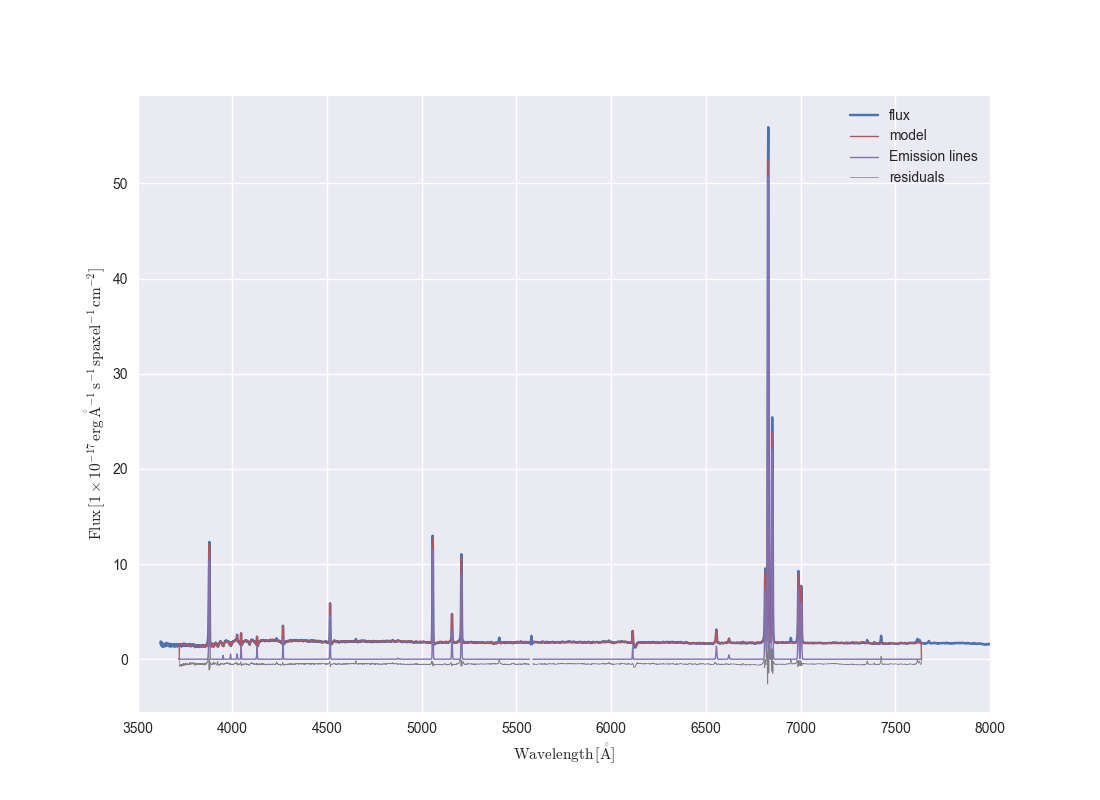Example of one binned spectrum in a MaNGA data cube. Lines show the binned flux, full model fit, model emission lines, and model fit residuals.

If we just want to compare the best fitting stellar continuum to the data, we should use the binned spectra within the DAP HYB10 LOGCUBE file:

dap_model_flux = my_bin.binned_flux
stellarcont_fit = my_bin.full_fit -  my_bin.emline_fit
ax = dap_model_flux.plot()
ax.lines.set_label('model flux')
ax.plot(stellarcont_fit.wavelength, stellarcont_fit.masked, color='g', lw=1, label='stellar cont.')
ax.legend()
ax.set_xlim(3500, 8000)
ax.set_ylim(1.2,2.2)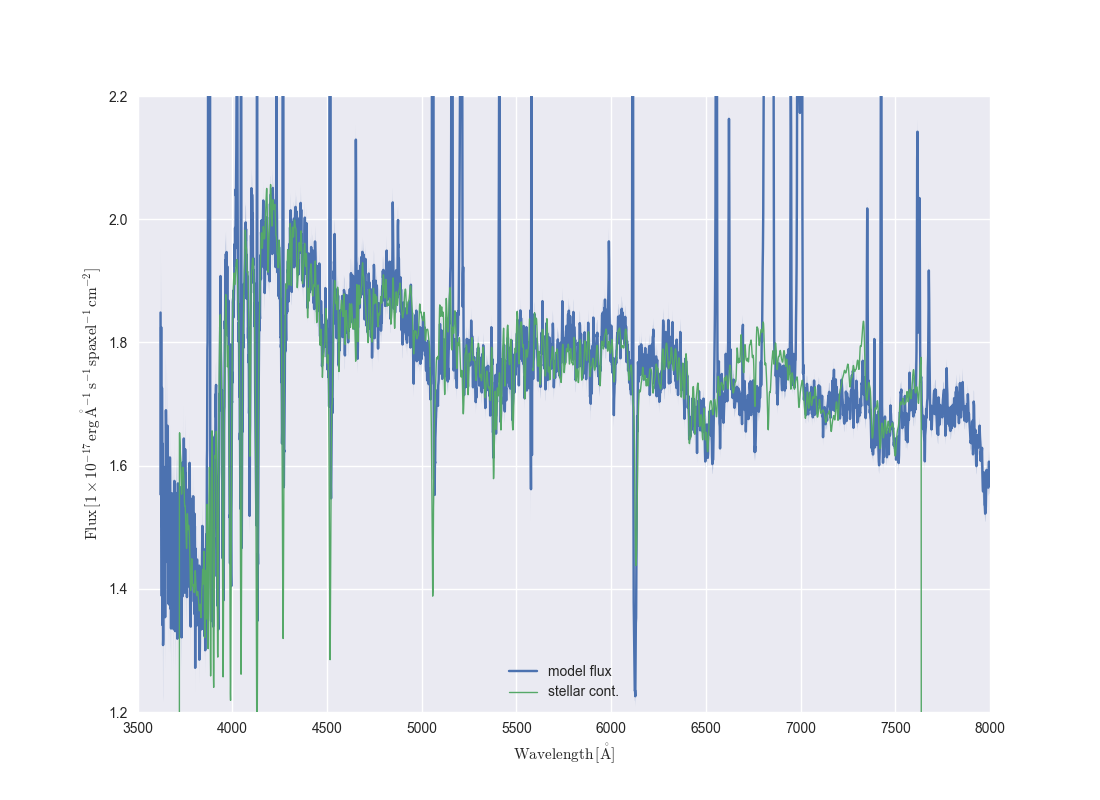HYB10 DAP stellar continuum plotted on top of the DAP binned flux.

You can find more tutorials in the Marvin documentation!